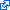## Contributed R Packages

R is a free softwareenvironment for statistical computing and graphics. R is distributed through CRAN (Comprehensive R Archive Network) mirrors. The analysis and code shown in the book are implemented by the following contributed packages:

### GeoModels 1.0.3-4: A Package for Geostatistical Gaussian and non Gaussian Data Analysis

This package provides a set of procedures for a) simulation and estimation of some spatial and spatio-temporal random fields using standard likelihood and a likelihood approximation method called composite likelihood, and b) prediction using best linear unbiased prediction. Spatio (temporal) bivariate data estimation involves estimation of both regression and covariance parameters.

Gaussian and some non Gaussian Random fields can be analyzed using the GeoModels package. Among them gamma, Weibull, log-Gaussian, binomial, negative binomial, skew Gaussian, Studend t and circular random fields can be analyzed.
Visit the project page on github### SpatialPack 0.3-8196: Tools for Assessment the Association Between Two Spatial Processes

This package provides tools to assess the association between two spatial processes. Currently, several methodologies are implemented: A modified t-test to perform hypothesis testing about the independence between the processes, a suitable nonparametric correlation coefficient, the codispersion coefficient, and an F test for assessing the multiple correlation between one spatial process and several others. Functions for image processing and computing the spatial association between images are also provided.
Visit the project page